Surface Area of Unit Sphere

Paradoxically, surface area first grows, and then starts to decrease as the number of dimensions goes over 7

In:=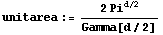In:=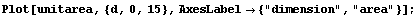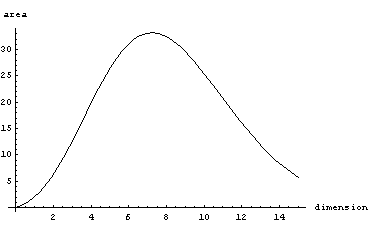Point of maximum surface area

In:=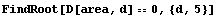Out=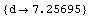Volume of Unit Sphere

In:=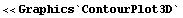In:=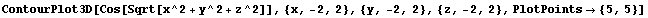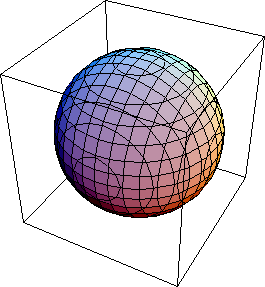Out=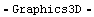Similar thing happens with volume of unit sphere

In:=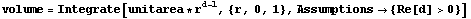Out=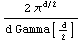In:=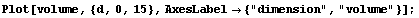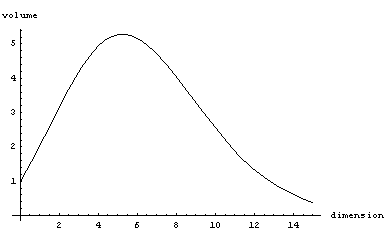In:=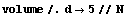Out=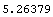Fraction in corners

As dimension grows, increasingly large fraction of mass is concentrated in the corners of unit cube (volume outside of the volume contained inside of an inscribed sphere)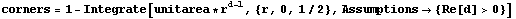Out=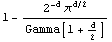In:=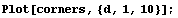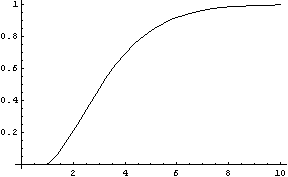In:=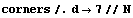Out=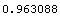Visualization of multidimensional cube

7 dimensional cube,most 96% of the mass is concentrated in"corners". For a d dimensional cube, square of the diagonal is d, so as the dimension increases, distance from the center of the cube, to the corners goes to infinity (whereas distance to any facet stays constant), so corners become long spikes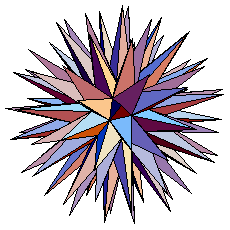E[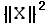] for standard Normal

For standard normal(identity covariance matrix, 0 mean), expected norm squared is just d (number of dimenions)

In:=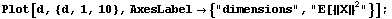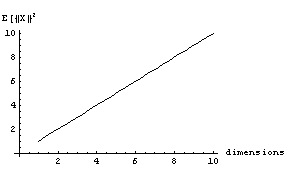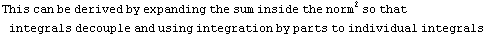E[{|X|}] for standard Normal

In:=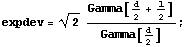In:=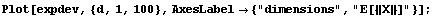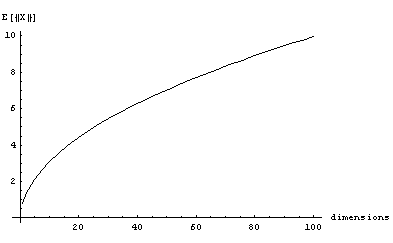This can be derived by converting to hyperspherical coordinates, and then using integration by parts/setting up a recurrence to integrate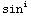x and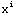exp(-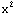)

Using the duplication formula for Gamma function we can rewrite

In:=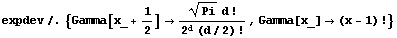Out=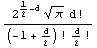Another simplification

In:=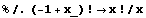Out=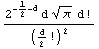Now we can use Stirling's approximation

In:=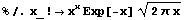Out=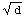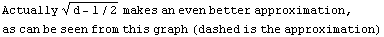In:=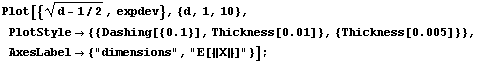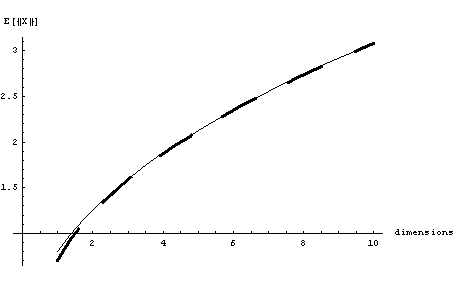Distribution of mass as function of radius for standard Normal

In:=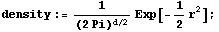In:=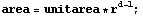In:=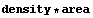Out=For one dimension, just right half of the standard normal

In:=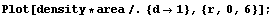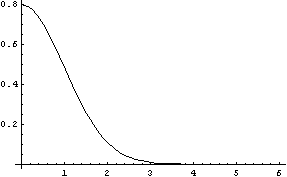For 4 and 16 dimensions, the mass starts to drift right

In:=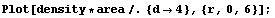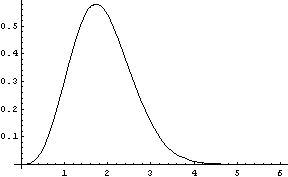In:=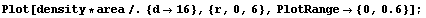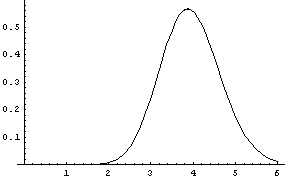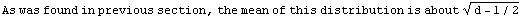We can use our expression for exact mean of the distribution of {|X|} to find the variance

In:=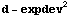Out=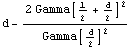In:=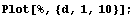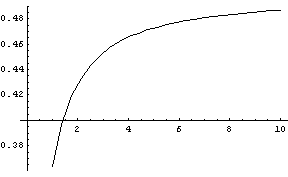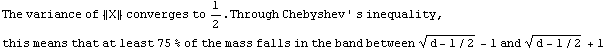Here's a numerical simulation to confirm this

In:=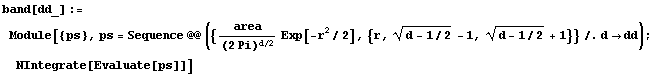In:=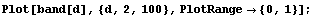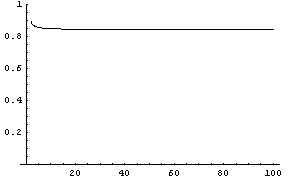In:=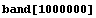Out=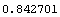It seems to converge to a value larger than predicted by Chebychev bound (.75) but much smaller than predicted using normality assumption (.95)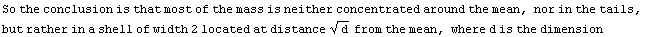Created by Mathematica  (May 18, 2006)